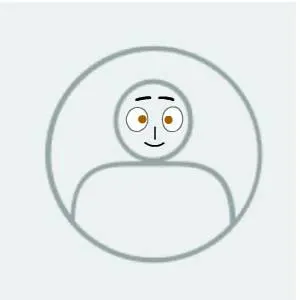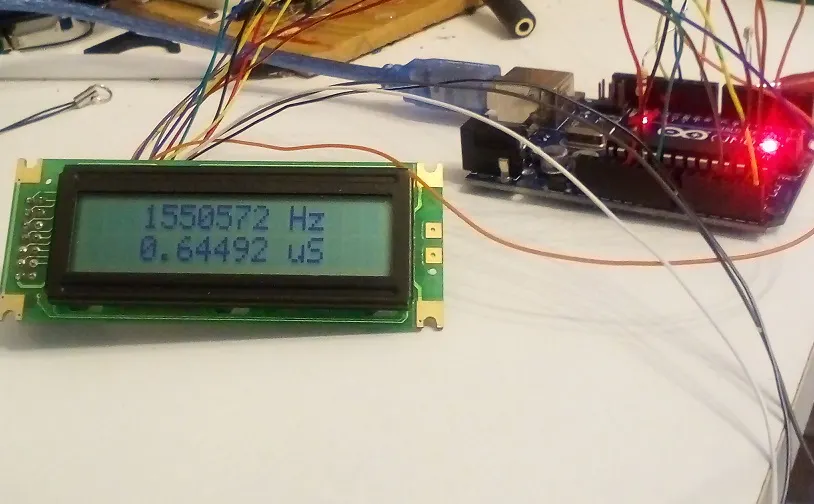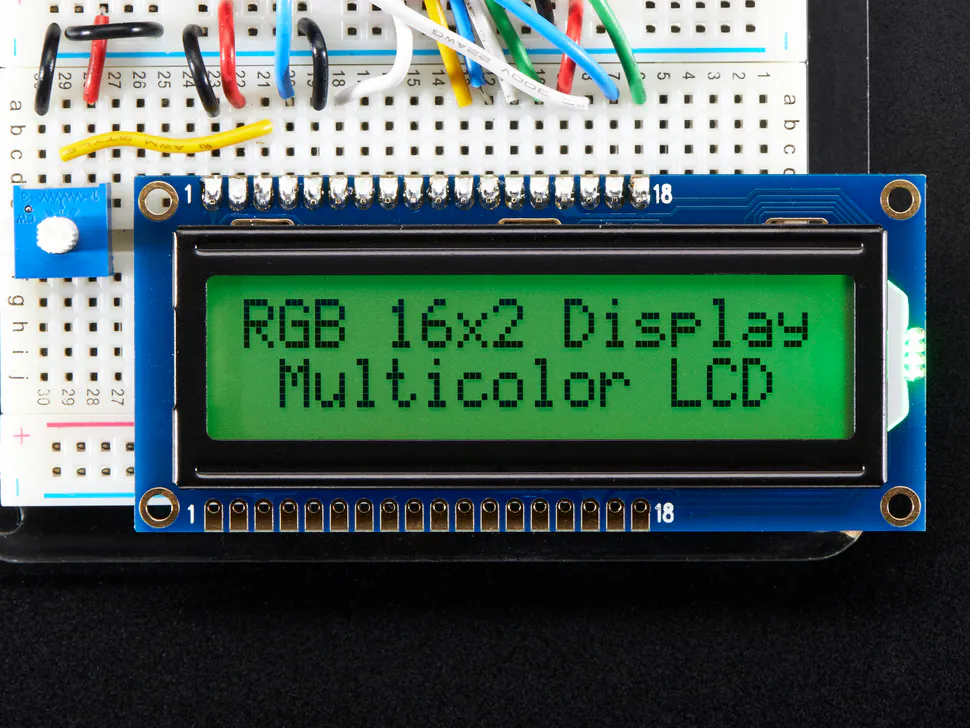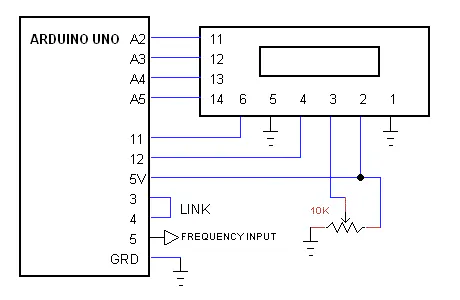Published

# Frequency Counter

Arduino Uno or Nano 6 MHz Frequency Counter with LCD. As accurate as the crystal.

BeginnerFull instructions provided8,029## Things used in this project

### Hardware componentsArduino UNO & Genuino UNO
×1Adafruit RGB Backlight LCD - 16x2
×1
 pot 10K
×1

## Schematics

### Counter## Code

### counter

C/C++
```/*
* LCD Counter
* Created: 12/08/2017 23:34:47
*  Author: moty22.co.uk
*/

#include <LiquidCrystal.h>

byte overF=0;
unsigned long freq;
double period;

// initialize the library with the numbers of the interface pins
LiquidCrystal lcd(12, 11, A2, A3, A4, A5);

// the setup function runs once when you press reset or power the board
void setup() {

lcd.begin(16, 2);   // set up the LCD's number of columns and rows:

//1 Hz timer
OCR0A = 249;
TCCR0A = _BV(WGM00) | _BV(WGM01) | _BV(COM0A0); //
TCCR0B = _BV(WGM02) | _BV(CS02) | _BV(CS01);  //  PWM mode, input T0 pin D4
pinMode(6, OUTPUT);

//250 Hz - timer2
OCR2A =249;
OCR2B = 125;
TCCR2A = _BV(COM2B1) | _BV(COM2B0) | _BV(WGM21) | _BV(WGM20);  //output B in phase, fast PWM mode
TCCR2B = _BV(WGM22) | _BV(CS22) | _BV(CS21); // set prescaler to 256 and start the timer
pinMode(3, OUTPUT);

//  counter input T1 pin D5
OCR1A = 32767;   //32768 counts
TCCR1A = _BV(WGM10) | _BV(WGM11) | _BV(COM1A0); //
TCCR1B =_BV(WGM12) | _BV(WGM13) | _BV(CS12) | _BV(CS11); //input pin D5
pinMode(9, OUTPUT);

lcd.setCursor(7, 0); // top middle
lcd.print("hi");

}

// the loop function runs over and over again forever
void loop() {

//wait for high
//start the count
TIFR1 = _BV(OCF1A);    //reset int
OCR1A = 32767;
TCNT1=0;
overF=0;
if(TIFR1 & (1<<OCF1A)) {++overF; TIFR1 = _BV(OCF1A);}   //overflow
}
//count end
freq = (unsigned long)TCNT1 + ((unsigned long)overF * 32768);
period = 1000000 / (double)freq;
if(freq == 0){period=0;}

lcd.clear();
lcd.setCursor(3, 0); // top line
lcd.print(freq,DEC);
lcd.setCursor(11, 0);
lcd.print("Hz");
lcd.setCursor(3, 1);
lcd.print(period,DEC);
lcd.setCursor(10, 1);
lcd.print(" uS   ");
}
```

## Credits

### moty

12 projects • 63 followers